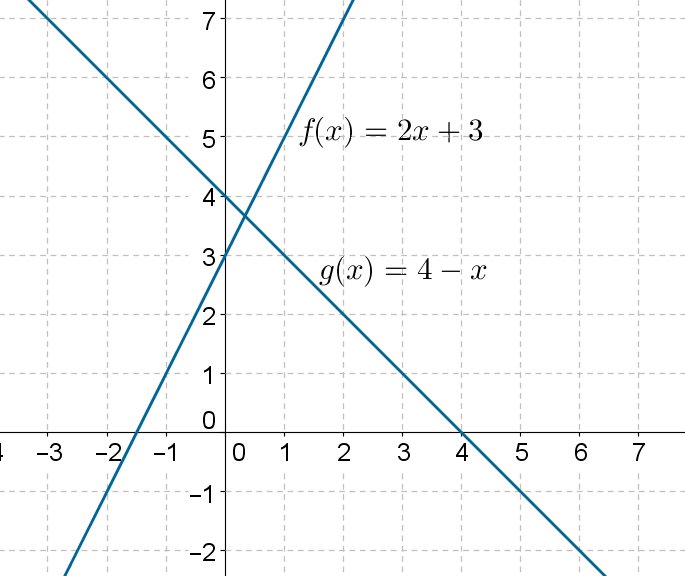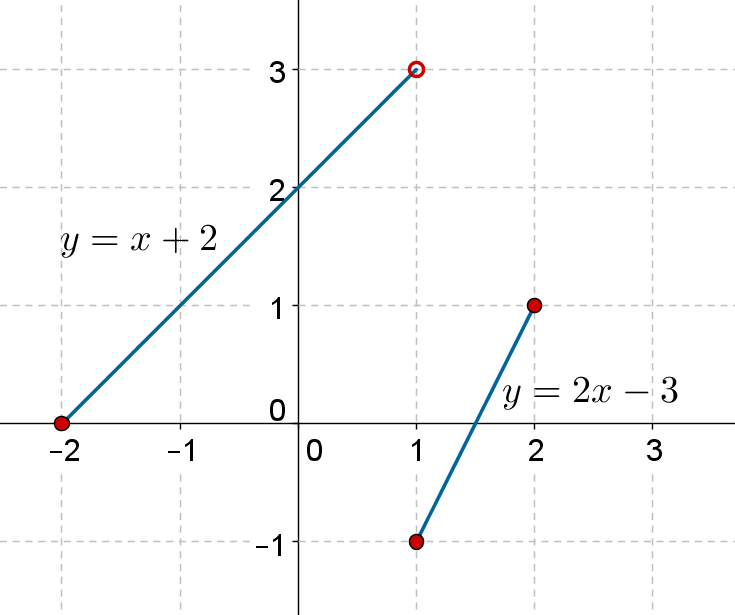# Linear Functions

Go back to  'Functions'

A linear function is a function of the form

$f\left( x \right) = ax + b,\,\,\,a \ne 0$

If a is 0, then we will think of f as a constant rather than as a linear function.

The domain of a linear function is the set of all real numbers, and so its range:

$D =\mathbb{R} ,\,\,\,R =\mathbb{R}$

The following figure shows $$f\left( x \right) = 2x+ 3$$ and $$g\left( x \right) = 4 - x$$ plotted on the same axes:Note that both functions take on real values for all values of x, which means that the domain of each function is the set of all real numbers. Look along the x-axis to confirm this. For every value of x, we have a point on the graph.

Also,the output for each function ranges continuously from negative infinity to positive infinity, which means that the range of either function is also $$\mathbb{R}$$ . This can be confirmed by looking along the y-axis, which clearly shows that there is a point on each graph for every y-value.

As we have seen in coordinate geometry, the slope of the line in the graph of $$f\left( x \right) = ax + b$$ will be $$m = a$$, and it will pass through the point $$\left( {0,b} \right)$$, because its y-intercept will be b.

Example 1: Plot the graph of

$f\left( x \right) = \left\{ \begin{array}{l}x + 2, & x \in \left[ { - 2,1}\right)\\2x - 3, & x \in \left[ {1,2}\right]\end{array} \right.$

Solution:This piecewise function is linear in both the indicated parts of its domain.The  graph is shown below:Functions
Functions
grade 10 | Questions Set 2
Functions
Functions
grade 10 | Questions Set 1